## 1) Using a line to indicate an axis

Extra axes can be added to a plot using the add_axis command, as will be shown in later examples. However, often all that is needed is a line, as used in this plot.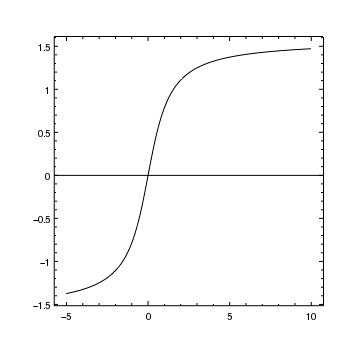Version: Postscript; PDF
```add_curve("atan.fits[cols X,Y]",["symbol.style","none"])

```

The simplest way to add an axis to a plot is to use add_hline or add_vline to draw a horizontal or vertical line, respectively. In this example add_hline is used to draw a line at y=0; this line will always extend across the whole plot, whatever the X-axis range is.

The settings for the current line can be found by using the get_line routine:

```chips> get_line()

color = default
depth = 100
extend = 3
id = None
stem = None
style = 1
thickness = 1.0

```

## 2) Adding an extra axis to a plot

In this example we create an actual axis, along with tick marks and labels, to indicate the y=0 position. Since it is a duplicate of the original axis, at the bottom of the plot, we bind together the axes so that they cover the same range. The following examples (A single plot containing two datasets with a common axis and Two y axes on the left of the plot) show how an extra axis can be used to create a new coordinate system, allowing different data to be added to the same plot.

The following plot extends this example to add an extra Y axis at x=0.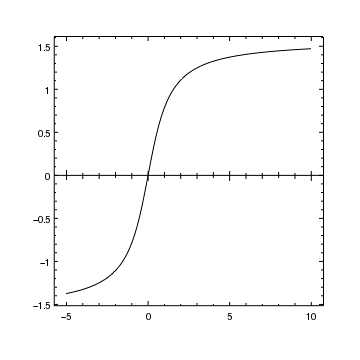Version: Postscript; PDF
```add_curve("atan.fits[cols X,Y]",["symbol.style","none"])

# The range of the axis is unimportant since the bind call
# will change it to match the existing X axis
bind_axes("ax1","ax2")

# Set the tick marks on the new axis to extend to both sides
axis = ChipsAxis()
axis.majortick.style = "centered"
axis.minortick.style = "centered"
axis.majortick.length = 10
axis.minortick.length = 6
axis.ticklabel.visible = False
set_axis("ax2",axis)
```

After creating the plot with add_curve, we add a second X axis at y=0 using add_axis. The first argument (X_AXIS) defines the axis type, the second argument the position (y=0), and the remaining arguments the initial range for this axis; in this example it doesn't matter what values we use, as explained below, so we pick 0 to 1. We specify that the location (i.e. y=0) is in the DATA coordinate system, otherwise it would refer to the plot-normalized system and so would place the new axis at the bottom of the plot.

The bind_axes call is used to make the new axis (which has been given the name ax2, as shown below in the info output) automatically mirror the original X axis range and scaling. After this call, a change to the scaling or limits of one axis will automatically be applied to the other.

Finally we adjust the length and placement of the tickmarks using the set_axis command, in order to extend to both sides of the axis (the default behavior is to just extend in one direction, as seen on the bottom and left-hand axes).

The info, info_current, info_coordinate and info_bound_axes commands provide information about the existing ChIPS objects; after creating the above plot the output of these commands looks like:

```chips> info()

Window [win1]
Frame [frm1]
Plot [plot1]   (0.15,0.15)  .. (0.90,0.90)
Border bottom [bx1]  top [bx2]  left [by1]  right [by2]
Curve [crv1]
X Axis [ax1]
Y Axis [ay1]
X Axis [ax2]

chips> info_current()

Window [win1]
Frame [frm1]
Plot [plot1]
Curve [crv1]
Y Axis [ay1]
X Axis [ax2]
Coord Sys [Data]
Coord Sys ID [ds0.0.0.4]

chips> info_coordinate()

Window [win1]
Frame [frm1]
Plot [plot1]
Coord Sys ID [ds0.0.0.3]
X Axis [ax1]
Y Axis [ay1]
Coord Sys ID [ds0.0.0.4]
X Axis [ax2]
Y Axis [ay1]

chips> info_bound_axes()

Window [win1]
Frame [frm1]
plot1:bx1   ->   plot1:bx2
plot1:by1   ->   plot1:by2
plot1:ax1   ->   plot1:bx2
plot1:ay1   ->   plot1:by2
plot1:ax1   ->   plot1:ax2

```

## 3) Adding extra axes to a plot

This is essentially the same as the Adding an extra axis to a plot example, except that we also add in an extra Y axis.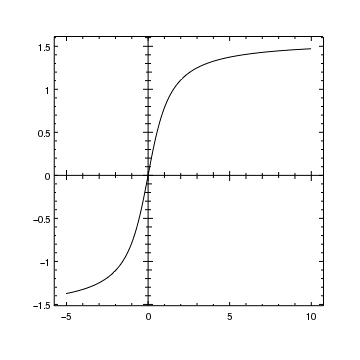Version: Postscript; PDF
```add_curve("atan.fits[cols X,Y]",["symbol.style","none"])

# The range of the axis is unimportant since the bind call
# will change it to match the existing X axis
bind_axes("ax1","ax2")

# Repeat for the Y axis
bind_axes("ay1","ay2")

# Set the tick marks on the new axis to extend to both sides
axis = ChipsAxis()
axis.majortick.style = "centered"
axis.minortick.style = "centered"
axis.majortick.length = 10
axis.minortick.length = 6
axis.ticklabel.visible = False
set_axis("ax2",axis)
set_axis("ay2",axis)
```

The info, info_current, info_coordinate and info_bound_axes commands provide information about the existing ChIPS objects; after creating the above plot the output of these commands looks like:

```chips> info()

Window [win1]
Frame [frm1]
Plot [plot1]   (0.15,0.15)  .. (0.90,0.90)
Border bottom [bx1]  top [bx2]  left [by1]  right [by2]
Curve [crv1]
X Axis [ax1]
Y Axis [ay1]
X Axis [ax2]
Y Axis [ay2]

chips> info_current()

Window [win1]
Frame [frm1]
Plot [plot1]
Curve [crv1]
X Axis [ax2]
Y Axis [ay2]
Coord Sys [Data]
Coord Sys ID [ds0.0.0.6]

chips> info_coordinate()

Window [win1]
Frame [frm1]
Plot [plot1]
Coord Sys ID [ds0.0.0.3]
X Axis [ax1]
Y Axis [ay1]
Coord Sys ID [ds0.0.0.5]
X Axis [ax1]
Y Axis [ay2]
Coord Sys ID [ds0.0.0.4]
X Axis [ax2]
Y Axis [ay1]
Coord Sys ID [ds0.0.0.6]
X Axis [ax2]
Y Axis [ay2]

chips> info_bound_axes()

Window [win1]
Frame [frm1]
plot1:bx1   ->   plot1:bx2
plot1:by1   ->   plot1:by2
plot1:ax1   ->   plot1:bx2
plot1:ay1   ->   plot1:by2
plot1:ax1   ->   plot1:ax2
plot1:ay1   ->   plot1:ay2

```

## 4) A single plot containing two datasets with a common axis

In this plot we want to display two sets of data with the same X axis (in this case time) but different Y axes (RA and Dec). This could be done using two plots, created using the strip_chart command - examples include Plotting related values in a strip chart and the plots of the Sharing an axis between plots section - but here we decide to use the same plot.

Since we have two different coordinate systems - (time, RA) and (time, Dec) - we need two Y axes; in this example we place the second axis on the right-hand side of the plot but it could go anywhere in the frame, as shown in the next example.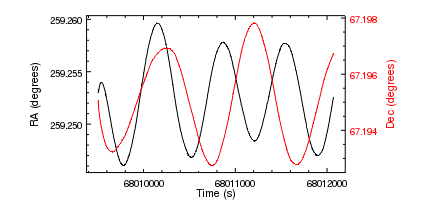Version: Postscript; PDF
```set_preference("curve.symbol.style","none")

set_plot_xlabel("Time (s)")
set_plot_ylabel("RA (degrees)")

# Place the second Y axis at the right-edge of the plot

# Since the new Y axis is now current, the new curve will be
# attached to its coordinate system and set the range
# of the axis automatically

set_plot_ylabel("Dec (degrees)")

set_yaxis(["label.color","red","ticklabel.color","red"])

# Note that both Y axes move as the plot is repositioned
# since they were positioned using the plot-normalized
# coordinate system
reposition_plot(0.2,0.2,0.8,0.93)

# We now change the attributes of the axes
ax = ChipsAxis()
ax.majortick.interval = 1e3
ax.tickformat = "%.0f"
ax.offset.perpendicular = 30
set_xaxis(ax)
ay = ChipsAxis()
ay.majortick.interval = 5e-3
ay.tickformat = "%.3f"
ay.minortick.count = 4
ay.offset.perpendicular = 60
set_yaxis("ay1",ay)
ay = ChipsAxis()
ay.majortick.interval = 2e-3
ay.tickformat = "%.3f"
ay.minortick.count = 3
ay.offset.perpendicular = 60
set_yaxis("ay2",ay)
set_axis("all",["majortick.mode","interval"])
```

We start by plotting the first dataset (time,RA) and setting up the axis labels. Note that by setting the id attribute of the curve (here to ra) in the add_curve call, we override the default name (crv1) that would normally be used; we do not actually make use of this name in this example, other than in the output of the various info commands.

We then add a second Y axis to the right-hand edge of the plot (x=1 in plot-normalized coordinates) using the add_axis command. Since we are unsure of the data range that will be used for this axis, we pick a simple range (here 0 to 1). The actual range is unimportant since it will be reset to match the data range in the add_curve call.

At this point - i.e. before the second add_curve call has been made - the output of the info, info_current, and info_coordinate commands looks like the following (note how the curve uses the name we gave, namely ra, rather than the default value of crv1):

```chips> info()

Window [win1]
Frame [frm1]
Plot [plot1]   (0.15,0.15)  .. (0.90,0.90)
Border bottom [bx1]  top [bx2]  left [by1]  right [by2]
Curve [ra]
X Axis [ax1]
Y Axis [ay1]
Y Axis [ay2]

chips> info_current()

Window [win1]
Frame [frm1]
Plot [plot1]
Curve [ra]
X Axis [ax1]
Y Axis [ay2]
Coord Sys [Data]
Coord Sys ID [ds0.0.0.4]

chips> info_coordinate()

Window [win1]
Frame [frm1]
Plot [plot1]
Coord Sys ID [ds0.0.0.3]
X Axis [ax1]
Y Axis [ay1]
Coord Sys ID [ds0.0.0.4]
X Axis [ax1]
Y Axis [ay2]
```

The current coordinate system is now "ds0.0.0.4" which - from the info_coordinate output - refers to the X axis and the second Y axis. The add_curve call therefore adds the data to this coordinate system, which results in the second Y axis changing to display the new data.

Since the second Y axis is current, the set_plot_ylabel and set_yaxis calls only change the right-hand Y axis. The plot is then re-positioned to ensure the axis labels can be moved further from the axes, so as to avoid overwriting the tick mark labels, without falling off the edge of the frame. The set_xaxis and set_yaxis calls are used to set these label offsets, as well as adjusting the location of the major tick marks. Note that specific axes can be targeted by giving the name of the axis as the first argument of the call - in this case "ay1" and "ay2". The final call uses the special name "all" to refer to all the axes in the current plot; alternatively the "majortick.mode" field of the ChipsAxis structure could have been set to "interval" in each of the preceeding three set_*axis calls.

The final ChIPS object hierarchy looks like the following: note that multiple Y axes are current due to the use of "all" as the identifier in the last set_axis call.

```chips> info()

Window [win1]
Frame [frm1]
Plot [plot1]   (0.20,0.20)  .. (0.80,0.93)
Border bottom [bx1]  top [bx2]  left [by1]  right [by2]
Curve [ra]
X Axis [ax1]
Y Axis [ay1]
Y Axis [ay2]
Curve [dec]

chips> info_current()

Window [win1]
Frame [frm1]
Plot [plot1]
Y Axis [by1]
Y Axis [by2]
X Axis [ax1]
Y Axis [ay1]
Y Axis [ay2]
Curve [dec]

```

## 5) Two y axes on the left of the plot

In the previous example we added the second Y axis to the right-hand edge of the plot. As we show below, the axis can be placed anywhere within the frame, even outside the plot.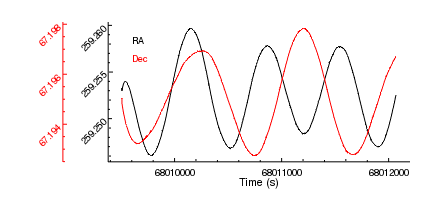Version: Postscript; PDF
```set_preferences(["curve.symbol.style","none","axis.offset.perpendicular",30])

reposition_plot(0.25,0.25,0.95,0.9)

set_xaxis(["majortick.interval",1e3,"tickformat","%.0f"])
ay = ChipsAxis()
ay.ticklabel.angle = 45
ay.majortick.interval = 5e-3
ay.tickformat = "%.3f"
ay.minortick.count = 4
set_yaxis(ay)

# Place the second Y axis to the left of the original axis

# Change the second Y axis to be red, with rotated axis labels
ay = ChipsAxis()
ay.all.color = "red"
ay.ticklabel.angle = 45
ay.majortick.interval = 2e-3
ay.tickformat = "%.3f"
ay.minortick.count = 3
ay.offset.perpendicular = 60
set_yaxis(ay)

# Change all the axes to use the interval mode
set_axis("all",["majortick.mode","interval"])

set_plot_xlabel("Time (s)")
```

Other than positioning the axis outside the plot, the only major differences to the previous example are:

1. Labels in the plot are used to indicate the data type, rather than axis labels. Note that these labels are positioned in data coordinates, which means that the Y values are very different for the two calls to add_label, namely 259.258 and 67.1965, even though they end up being displayed close to each other on the plot.

An alternative would have been to place the labels in plot-normalized coordinates by saying:

```add_label(0.1, 0.85, "RA", ["coordsys", PLOT_NORM])
add_label(0.1, 0.75, "Dec", ["coordsys", PLOT_NORM, "color", "red"])
```
2. The use of all.color in the set_curve call to set all the *.color attributes of the second Y axis - such as label.color and majortick.color - to the value "red".

## 6) Creating axes using a WCS transform

In this example we show how you can create a pair of axes that display a tangent-plane projection, in this case read in from a file. It also shows how axes can be positioned outside the plot, and bound together.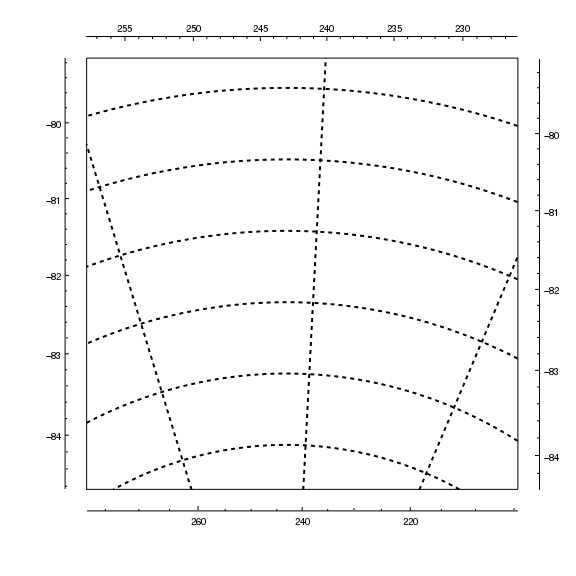Version: Postscript; PDF
```add_window(8,8,"inches")

# Read in the file with the WCS-Tan projection we wish to use,
# and extract the details of this transformation
tr = cr.get_transform('EQPOS')

# Create a pair of axes using this transform, picking
# axis limits that are roughly correct (these will be
# changed by the set_data_aspect_ratio)
add_axis(XY_AXIS, -0.05, 255, 235, -85, -80, tr)
set_data_aspect_ratio("1:1")

# Turn on the major grid lines and hide the borders
set_axis(["majorgrid.visible",True,"majorgrid.thickness",2])
set_plot(["style","boxed"])

# Zoom out a bit
zoom(0.4)

# Create another axis pair, this time on the top/right of the plot
# and ensure they are bound to the original axes
add_axis(XY_AXIS, 1.05, 255, 235, -85, -80, tr)
bind_axes("ax1","ax2")
bind_axes("ay1","ay2")
```

We use the Crates read_file routine to read in the file, and the get_transform method to get the details of the WCS tangent-plane transformation. The transform - tr - is passed to the add_axis call to create a pair of axes that display a tangent-plane, rather than cartesian, projection (the first arguemnt must be XY_AXIS in this case as both axes have to be created at the same time).

We could have created the axes at x/y = 0 and then used move_axis to move them to -0.05, i.e. just outside the plot area. In this case, since no ChipsId structure was used to set the coordinate system, the axes are positioned using the plot-normalized system.

The aspect ratio is set, using the set_data_aspect_ratio command, so that both axes have the same scale. This adjusts the limits that were set in the add_axis call.

After turning on the major grid lines, and zooming out the plot slightly, we add another pair of axes, this time to the top and right of the plot, and bind these tot he existing axes so that they match. Since we have a non-cartesian projection, they do not cover the same range (this is particularly noticeable for the two X axes).

Note that the tick marks are drawn at the correct position for the axis given that it has been moved away from the plot edge; for example the Y axis major tick marks do not line up vertically at the position where the dotted lines reach the edge of the plot, nor to the X axis marks line up horizontally with the corresponding grid lines.